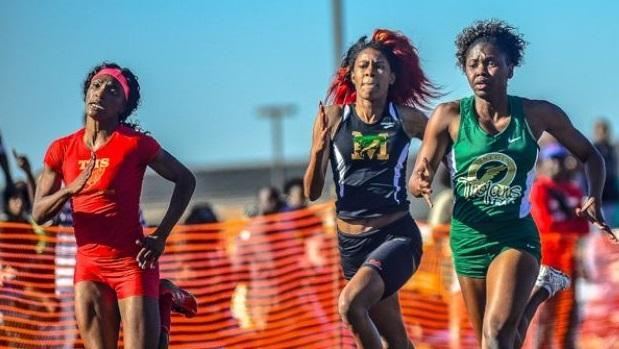# 2A Girls Team Power Rankings Thru 4-2

###Mahogony Walter from Thomasville will be a threat to triple in the 100,200 and HJ this season

### 2015 ga HS F Outdoor Track Team Scores

Calculated as of April 03, 2015

These rankings were calculated by scoring the rankings as a meet. We scored 10 deep for individuals and 10 deep for relays. For example, for individuals 1st place received 10 points for their team, on down to 1 point for 10th place.

1.Lovett111.75
Emma Sidman (1600m 3 = 3)
Khadejah Jackson (300H 10 + TJ 7 = 17)
Megan McGarrity (800m 6 = 6)
Nicole Teague (100m 10 + LJ 9 + TJ 10 = 29)
Relay Team (17)
Sarah Carpenter (PV 0.75 = 0.75)
Serena Tripodi (1600m 10 + 3200m 10= 20)
Victoria Powell (200m 10 + 400m 9 = 19)
2.Greater Atlanta Christian100
Abby Kettle (1600m 6 + 3200m 8 = 14)
Charissa DAvis (800m 1 = 1)
Danielle Ship (HJ 7 = 7)
Jada Jones (100m 7 + 100H 6 = 13)
Kennedy Street (200m 6 + 400m 8 = 14)
Maria Harris (D 3 = 3)
Nia Brown (100m 6 + 200m 4 = 10)
Relay Team (17)
Ripley Fricano (1600m 4 = 4)
Stephanie Reynolds (100H 10 + HJ 7 = 17)
3.Thomasville High School94.5
Aleah Wagner (100H 8 + 300H 5 = 13)
Danisha Jones (D 10 + S 10 = 20)
Demetria Miley (800m 5 = 5)
Mahogony Walter (100m 9 + 200m 9 + HJ 9 = 27)
Miyah Beard (800m 3.5 = 3.5)
Naomi Toles (100m 1 + TJ 8 = 9)
Relay Team (15)
Sherriona Jackson (LJ 2 = 2)
4.Wesleyan School75.75
Adrienne Lee (PV 7 = 7)
Cairo Booker (200m 5 + 400m 3 = 8)
Ellie Bradach (1600m 7 + 3200m 7 = 14)
Georgia Kuehn (PV 0.75 = 0.75)
Jayla Jones (300H 2 = 2)
Jordan Zimmerman (1600m 2 = 2)
Kylie Reed (800m 7 = 7)
Lauren Frerking (D 2 = 2)
Maddie Jones (HJ 3 = 3)

Nikki Villa (3200m 5 = 5)
Relay Team (13)
Sheridan Davenport (200m 7 + 400m 5 = 12)

5.Jeff Davis76.75
Hannah Mason (HJ 3 = 3)
La'Tonya Smith (S 3 = 3)
Mariah Gibbs (D 4 + S 7 = 11)
Morgan Carelock (300H 7 + 400m 7 + HJ 10 = 24)
Relay Team (22)
Taylor Hosmer (PV 0.75 = 0.75)
Tiana Hansley (HJ 3 = 3)
Tristan Herndon (D 8 + S 2 = 10)
6.Northeast Macon61.5
Alexis Mitchell (200m 1 + 400m 2 = 3)
Jaliah Jackson (300H 6 + LJ 4 + TJ 5 = 15)
Kamare Wayes (100H 9 + 300H 8 + TJ 4 = 21)
Relay Team (16)
Tiyanna Lester (LJ 6.5 = 6.5)
7.Bremen High School55.5
Andrea Lasseigne (HJ 7 = 7)
Caroline Brown (D 6 + S 1 = 7)
Katie Hanegan (PV 8 = 8)
Kaylee Riley (PV 9.5 = 9.5)
Relay Team (8)
Zharia Johnson (100H 7 + 300H 3 + TJ 6 = 16)

8.Darlington School50.5

Kate Flory (3200m 2 = 2)
Kinslee Clevenger (1600m 1 + 800m 3.5 = 4.5)
Lauren Hooper (3200m 6 = 6)
Paige Mavity (PV 6 = 6)
Relay Team (13)
Stephanie Ward (1600m 9 + 800m 10 = 19)

 8. Bleckley County High School 46 Alondria Clemons (100H 2 = 2) Ellen Powell (3200m 9 + 800m 8 = 17) Relay Team (14) T'Keyah Pasby (D 5 + S 8 = 13)

Taylor DeLoach (100m 8 + 200m 8 + LJ 10 = 26)
11.Gordon Lee High School25.75
Addison Whitman (PV 0.75 = 0.75)
Anna Pettigraw (PV 3 = 3)
Madelyn Lee (D 7 + S 9 = 16)
Shay Wooten (S 6 = 6)
12.Pelham High School24.5
Amelia Almond (D 9 + S 5 = 14)
Cheyenne Brooks (PV 9.5 = 9.5)
Mahogany Brown (TJ 1 = 1)
Julia Ross (800m 2 = 2)
Lacey O'sullivan (1600m 8 = 8)
Lauren Hadley (300H 4 + LJ 8 = 12)
Relay Team (1)
Sophie Blasberg (300H 1 = 1)
14.Holy Innocents' Episcopal School22
Chelsea Zoller (300H 9 + 400m 10 = 19)
Parke Faison (400m 1 = 1)
Relay Team (2)
15.Fitzgerald20
Alex Wallace (PV 4.5 = 4.5)
Bethany Clark (PV 4.5 = 4.5)
Kirsten Crook (100H 1 + LJ 1 = 2)
Markeela Bozeman (LJ 5 = 5)
Relay Team (4)
16.Oglethorpe County HS12
Kiana Jackson (200m 2 = 2)
Trenetta Smith (100H 5 + LJ 3 + TJ 2 = 10)
17.Swainsboro High School10
Carianna Troupe (TJ 9 = 9)
Kalen Wadley (D 1 = 1)
18.East Laurens9.5
J'Mya Canady (LJ 6.5 + TJ 3 = 9.5)
19.Washington-Wilkes High School9
D'Meka Davis (100m 3 = 3)
Relay Team (6)
20.Manchester6
Ternazha Mcgruder (400m 6 = 6)
21.Temple High School5
Khadijah Montgomery (100m 5 = 5)
22.Berrien County HS4
Treyonna Boone (S 4 = 4)
22.Monticello4
Kamia Thomas (100H 4 = 4)
24.Bryan County HS3
Lianna Shuman (HJ 3 = 3)